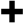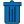XF's Debye-Drude material definition offers two dispersive models—Debye and Drude—whose complex permittivity varies with frequency. The models support multiple poles for more flexibility when fitting the XF material to a set of measured data. Because XF uses the finite-difference time-domain (FDTD) method, a single simulation includes this dispersion over a range of frequencies, for example 850 MHz to 6 GHz.

Debye vs Drude

XF's Debye-Drude material definition specifies either a Debye material or a Drude material. The presence of a conductivity term separates the two models and leads to different complex permittivity curves as shown in the following graph.The Debye material's complex relative permittivity versus frequency, $\tilde\epsilon(\omega)$, is described by an equation with one or more first order poles. For a single pole, it is written as

\begin{equation} \tilde\epsilon(\omega) = \epsilon_\infty + \frac{\epsilon_s - \epsilon_\infty}{1 + j\omega\tau_0} \end{equation}

where $\epsilon_s$ is the static relative permittivity, $\epsilon_\infty$ is the infinite frequency relative permittivity, $\omega$ is the angular frequency, and $\tau_0$ is the relaxation time. The graphs shows that $\epsilon_s$ is the relative permittivity at DC or 0 Hz, $\epsilon_\infty$ is the relative permittivity at higher frequencies, and the transition between them is controled by $\omega$ and $\tau_0$.

XF's support of n poles expands the model to

\begin{equation} \tilde\epsilon(\omega) = \epsilon_\infty + \frac{\epsilon_{s1} - \epsilon_\infty}{1 + j\omega\tau_1} + \frac{\epsilon_{s2} - \epsilon_\infty}{1 + j\omega\tau_2} + ... + \frac{\epsilon_{sn} - \epsilon_\infty}{1 + j\omega\tau_n} \end{equation}

The Drude dispersion relation takes the same form as the Debye equation, but includes a lossy term. A non-zero conductivity term, $\sigma$, generates a Drude material, written as

\begin{equation} \tilde\epsilon(\omega) = \epsilon_\infty + \frac{\epsilon_s - \epsilon_\infty}{1 + j\omega\tau_0} + \frac{\sigma}{j\omega\epsilon_0} \end{equation}

where $\epsilon_0$ is the free space permittivity of 8.854e-12 F/m. XF's Drude model can also be extended with multiple poles for flexibility when fitting measured material data. It is described as

\begin{equation} \tilde\epsilon(\omega) = \epsilon_\infty + \frac{\epsilon_{s1} - \epsilon_\infty}{1 + j\omega\tau_1} + \frac{\epsilon_{s2} - \epsilon_\infty}{1 + j\omega\tau_2} + ... + \frac{\epsilon_{sn} - \epsilon_\infty}{1 + j\omega\tau_n} + \frac{\sigma}{j\omega\epsilon_0} \end{equation}

Material EditorDebye and Drude material parameters are available in the Material Editor once the Type is set to Debye-Drude under the Electric tab. The available fields relate to the terms above and theandbuttons add and remove poles, respectively. Although the editor does not display complex permittivity versus frequency, the Plot Material Parameters macro will graph material properties.

Clicking the Dispersive Material Calculator button opens a separate window where users can fit an XF material to measured data.

The Infinite Dielectric Strength option applies to electrostatic discharge (ESD). In order to perform this analysis, users must uncheck the box and provide a finite dielectric strength value. This parameter does not factor into Maxwell's equations for computing electric and magnetic fields; it is only used to detect possible dielectric breakdown in ESD analysis.